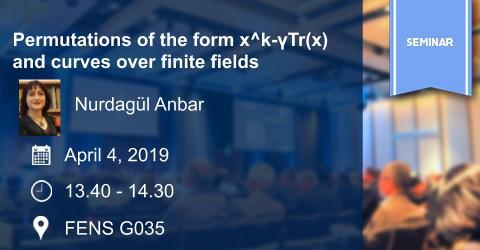## Algebra Seminar: Permutations of the form x^k-γTr(x) and curves over..

### Algebra Seminar: Permutations of the form x^k-γTr(x) and curves over..Speaker: Nurdagül Anbar

Title: Permutations of the form x^k-γTr(x) and curves over finite fields

Date/Time: April 4, 2019  /  13.40-14.30

Place: FENS G035

Abstract:  Let q be a power of a prime p, and let Fq be the finite field with q elements. A polynomial P(x) ∈ Fq[x] is called a permutation of Fq if the associated map from Fq to Fq defined by x 7→ P(x) is a bijection, i.e., it permutes the elements of Fq. In this talk, we consider the polynomials of the form P(x) = x k − γTr(x) over Fqn for n ≥ 2, where Fqn is the extension of Fq of degree n and Tr is the absolute trace from Fqn to Fq. We show that P(x) is not a permutation of Fqn in the case gcd(k, qn − 1) > 1. Our proof uses an absolutely irreducible curve over Fqn and the number of rational points on it.

Contact:  Michel Lavrauw